The NPAR1WAY Procedure

Scores for Linear Rank and One-Way ANOVA Tests

For each score type that you specify, PROC NPAR1WAY computes a one-way ANOVA statistic and also a linear rank statistic for two-sample data. The following score types are used primarily to test for differences in location: Wilcoxon, median, Van der Waerden (normal), and Savage. The following scores types are used to test for scale differences: Siegel-Tukey, Ansari-Bradley, Klotz, and Mood. Conover scores can be used to test for differences in both location and scale. This section gives formulas for the score types available in PROC NPAR1WAY. For further information about the formulas and the applicability of each score, see Randles and Wolfe (1979); Gibbons and Chakraborti (2010); Conover (1999); Hollander and Wolfe (1999).

In addition to the score types described in this section, you can specify the SCORES=DATA option to use the input data observations as scores. This enables you to produce a wide variety of tests. You can construct any scores by using the DATA step, and then you can use PROC NPAR1WAY to compute the corresponding linear rank and one-way ANOVA tests for these scores. You can also analyze raw (unscored) data with the SCORES=DATA option; for two-sample data, the corresponding exact test is a permutation test that is known as Pitman’s test.

Wilcoxon Scores

Wilcoxon scores are the ranks of the observations, which can be written as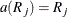where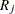is the rank of observation j, and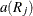is the score of observation j.

Using Wilcoxon scores in the linear rank statistic for two-sample data produces the rank sum statistic of the Mann-Whitney-Wilcoxon test. Using Wilcoxon scores in the one-way ANOVA statistic produces the Kruskal-Wallis test. Wilcoxon scores are locally most powerful for location shifts of a logistic distribution.

When computing the asymptotic Wilcoxon two-sample test, PROC NPAR1WAY uses a continuity correction by default, as described in the section Continuity Correction. If you specify the CORRECT=NO option in the PROC NPAR1WAY statement, the procedure does not use a continuity correction.

Median Scores

Median scores equal 1 for observations greater than the median, and 0 otherwise. In terms of the observation ranks, median scores are defined as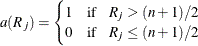Using median scores in the linear rank statistic for two-sample data produces the two-sample median test. The one-way ANOVA statistic with median scores is equivalent to the Brown-Mood test. Median scores are particularly powerful for distributions that are symmetric and heavy-tailed.

Van der Waerden (Normal) Scores

Van der Waerden scores are the quantiles of a standard normal distribution and are also known as quantile normal scores. Van der Waerden scores are computed as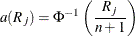where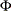is the cumulative distribution function of a standard normal distribution. These scores are powerful for normal distributions.

Savage Scores

Savage scores are expected values of order statistics from the exponential distribution, with 1 subtracted to center the scores around 0. Savage scores are computed as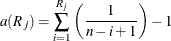Savage scores are powerful for comparing scale differences in exponential distributions or location shifts in extreme value distributions (Hajek, 1969, p. 83).

Siegel-Tukey Scores

Siegel-Tukey scores are defined as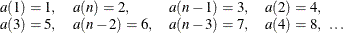where the score values continue to increase in this pattern toward the middle ranks until all observations have been assigned a score.

When computing the asymptotic Siegel-Tukey two-sample test, PROC NPAR1WAY uses a continuity correction by default, as described in the section Continuity Correction. If you specify the CORRECT=NO option in the PROC NPAR1WAY statement, the procedure does not use a continuity correction.

Ansari-Bradley scores are similar to Siegel-Tukey scores, but Ansari-Bradley scoring assigns the same score value to corresponding extreme ranks. (Siegel-Tukey scores are a permutation of the ranks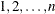.) Ansari-Bradley scores are defined as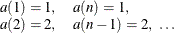Equivalently, Ansari-Bradley scores are equal to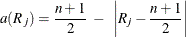Klotz Scores

Klotz scores are the squares of the Van der Waerden (normal) scores. Klotz scores are computed as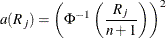whereis the cumulative distribution function of a standard normal distribution.

Mood Scores

Mood scores are computed as the square of the difference between the observation rank and the average rank. Mood scores can be written as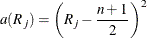Conover Scores

Conover scores are based on the squared ranks of the absolute deviations from the sample means. For observation j the absolute deviation from the mean is computed as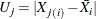where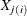is the value of observation j, observation j belongs to sample i, and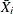is the mean of sample i. The values of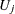are ranked, and the Conover score for observation j is computed as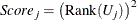Following Conover (1999), when there are ties among the values of, PROC NPAR1WAY assigns the average rank to each of the tied observations. To compute the average rank, PROC NPAR1WAY ranks theas if there were no ties, and then averages the ranks of the tied observations.

The Conover score test is also known as the squared ranks test for variances. See Conover (1999) for more information.